# Non-Pascalean geometry

(diff) ← Older revision | Latest revision (diff) | Newer revision → (diff)
A geometry with a non-commutative multiplication. As a consequence of the fact that in affine geometry the property of commutativity is equivalent to the Pascal theorem, the name non-Pascalean geometry is usually attached to a geometry in which the following theorem fails to hold: Suppose that on each of two intersecting straight lines three points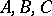andare given, other than the point of intersection of the lines; ifis parallel to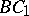and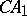is parallel to, then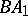is parallel to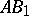. This is sometimes called Pappus' theorem; it is a special case of the theorem of Pascal in the theory of conic sections (namely, when the conic degenerates to a pair of straight lines).